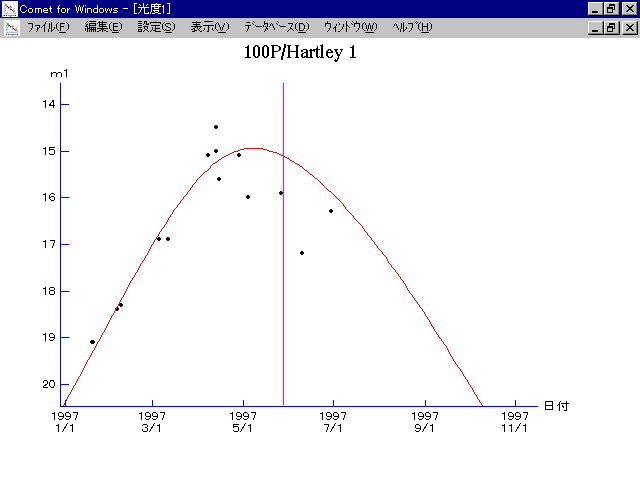# \$B%O!<%H%l!

100P/Hartley 1 (1997)###\$B50F;MWAG(B

```   The following improved orbital elements by Kenji Muraoka, are
from 100 observations 1985 to 1997, perturbations by 9 Plantes,
Moon and 5 minor planets were taken into account.
The mean residual is +/- 0.78 arc seconds.

Epoch  =  1997 June  1.0  TT       JDT = 2450600.5
T  =  1997 May  28.51692       +/- 0.00009 (m.e.) TT
Peri. =  178.93279                +/- 0.00004
Node  =   38.90298                +/- 0.00006   (2000.0)
Incl. =   25.72329                +/- 0.00005
q  =    1.8186831              +/- 0.0000032 AU
e  =    0.4506090              +/- 0.0000008
a  =    3.3103621              +/- 0.0000023 AU
n  =    0.16364051             +/- 0.00000017
P  =    6.023                  +/- 0.0000063  years
```

###\$B@1?^(B1996\$BG/(B11\$B7n(B 3\$BF|!A(B1997\$BG/(B12\$B7n(B28\$BF|(B

###\$B8wEYJQ2=(B

```        m1 = 2.0 + 5 log\$B&\$(B + 50.0 log r
```##### \$B50F;MWAG\$OB<2,7r<#;a\$N7W;;\$K\$h\$k\$b\$N\$G\$9!#(B \$B@1?^\$O(B StellaNavigator Ver.2.0 for Windows (\$B%"%9%H%m%"!<%D(B \$BJTCx(B / \$B%"%9%-!<=PHG6I4)(B) \$B\$G:n@.\$7\$?\$b\$N\$G\$9!#(B \$B8wEY%0%i%U\$O(BComet for Windows\$B\$G:n@.\$7\$?\$b\$N\$G\$9!#(B